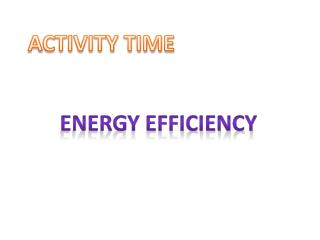DownloadDownload PresentationENERGY EFFICIENCY

# ENERGY EFFICIENCY

Télécharger la présentation## ENERGY EFFICIENCY

- - - - - - - - - - - - - - - - - - - - - - - - - - - E N D - - - - - - - - - - - - - - - - - - - - - - - - - - -
##### Presentation Transcript

1. ACTIVITY TIME ENERGY EFFICIENCY
2. Before we start with the activity some basic terms are explained here: Wattage (w): Mentioned in the equipment for e.g. A 40 Watt bulb, an 18watt CFL etc. Kilowatt (Kw): When the wattage is divided by 1000, you get Kw. e.g. The Kw of a 40 w bulb is 40/1000 Kw and for an 18 w CFL is 18/1000 Kw. Kilowatt Hour (KwH): When the Kw is multiplied by the hours of usage of the equipment what we achieve is known as KwH. For e.g. if we use the 18 W CFL bulb for 8 hours a day, the KwH for an 18 W CFL will be (18/1000 x 8 hours). KwH for a Month will be (18/1000 x 240 hours{8 hrs. x 30 days a month})
3. Calculation of Electricity consumption per Month Watt/1000 X Running Hours per Month = Units Consumed/ Month * Days per Month : 30 * Running Hours : Depends on the usage of the equipment
4. A CASE STUDY Ram and Rahim are neighbours. They both have identical houses and electrical usages. Even after having so much similarity, it was found that Ram’s electricity bill was way higher than that of Rahim’s. This led Ram to introspect what Rahim did to save his electricity consumption.
5. Now let us study the usage of electricity of Ram
6. Now let us study the usage of electricity of Rahim
7. What is your conclusion? A) Units saved per month by Rahim = ________ B) Units saved per year (A x 12) = __________ Total saving of Rahim per annum = units saved x unit charge = Rs ______________ Assume Unit Charge = Rs 5.40. Let us round it to Rs 5.00
8. Rahim’s Investment
9. Ram’s Investments
10. Let us now calculate Rahim’s Payback period Rahim invested = Rs ___________ more His savings per annum= Rs ______________ Recovery period in years = ___________years (Invested amount / Savings)
11. Conclusion Rahim recovers his extra investment in _____ years, and continues to save Rs ____ every year, but Ram has to go on paying Rs _____ more every year because he has not used Star Labeled equipments.
12. THANK YOU